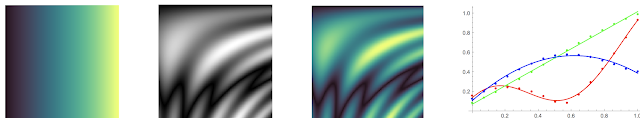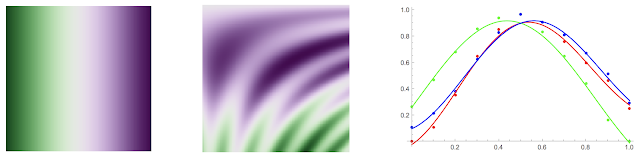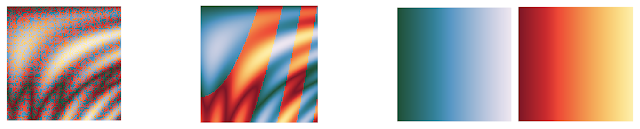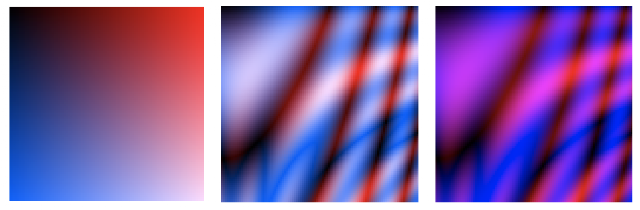## 18 November, 2017

### "Coder" color palettes for data visualization

Too often when programmers want to visualize data (which they should do often!), we simply resort to so called "coder-colors", encoding values directly into RGB channels (e.g. R = data1, G = data2 ...) without much consideration.

This is unfortunate, because it can both significatively distort the data, rendering it in a non perceptually linear fashion and biasing certain data columns to be more important than others (e.g. the blue channel is much less bright than the green one), and make the visualization less clear as we leverage only one color characteristic (brightness) to map the data.

The idea here is to build easy to use palette approximations for data visualization that can be coded as C/Java/Shader/etc... functions and replace "coder colors" with minimal effort.

Features we're looking for:

• Perceptual linearity
• The palette steps should be equal in JND units
• We could prove this by projecting the palette in color space made for appearance modeling (e.g. CIELAB) and looking at the gradient there.
• Good range
• We want to use not just brightness, but color variations as well.
• We could even follow curved paths in a perceptually linear color space, we are not restricted to straight lines..
• The objective is to be able to clearly distinguish >10 steps.
• Intuitive for the task at hand, legible
• E.g sequential data (0...1) versus diverging or categorical data (-1...1).
• Colorblind aware
• The encoding should primarily rely on brightness variation, color variation should be used only to try to increment the range/contrast and using colorblind safe colors.
Now, before I dump some code, I have to disclaim that albeit I tried to follow the principles listed above, I don't claim I am absolutely confident in the end results... Color appearance modelling is quite hard in practice, it depends on the viewing environment and the overall image being displayed, and there are many different color spaces that can be used.

The following palettes were done mostly by using CIELAB ramps and/or looking at well-known color combinations used in data visualization.
The code below is GLSL, but I avoided on purpose to use GLSL vectors so it's trivial to copy and paste in C/Java/whatever else...

One-dimensional data.

vec3 ColorFn1D (float x)
{
x = clamp (x, 0.0, 1.0);
float r = -0.121 + 0.893 * x + 0.276 * sin (1.94 - 5.69 * x);
float g = 0.07 + 0.947 * x;
float b = 0.107 + (1.5 - 1.22 * x) * x;
return vec3 (r, g, b);
}

This palette is similar to R's "Viridis", even if it wasn't derived from the same data. You can notice the sine in one of the channels, it's not unusual for most of these palettes to be well approximated using sine waves because the most straightforward way to derive a brighness-hue-saturation perceptual color space is to use cylindrical transforms of color spaces that are rotated so one axis represents brightness, and the other two are color components (e.g. that's how CIELAB works with the related cylindrical transforms like CIELCH and HSLUV)Palette, example use and sRGB plot

Note how the palette avoids stretching to pure black. This is wise both because the bottom range of sRGB is not great in terms of perceptual uniformity, and because lots of output devices won't do particularly great when dealing with blacks.

One-dimensional data, diverging.

vec3 ColorFn1Ddiv (float y)
{
y = clamp (y, -1.0, 1.0);
#if 0
float r = 0.569 + (0.396 + 0.834 * y) * sin (2.15 + 0.93 * y);
float g = 0.911 + (-0.06 - 0.863 * y) * sin (0.181 + 1.3 * y);
float b = 0.939 + (-0.309 - 0.705 * y) * sin (0.125 + 2.18 * y);
#else
float r = 0.484 + (0.432 - 0.104 * y) * sin(1.29 + 2.53*y);
float g = 0.334 + (0.585 + 0.00332 * y) * sin(1.82 + 1.95*y);
float b = 0.517 + (0.406 - 0.0348 * y) * sin(1.23 + 2.49*y);
#endif
return vec3 (r, g, b);
}Palette, example use and sRGB plot

One-dimensional data, two categories.

Essentially, one dimensional data + a flag. It choses between two palettes that are designed to be similar in brightness but always quite easy to distinguish, at any brightness level.

vec3 ColorFn1DtwoC (float x, int c)
{
x = clamp (x, 0.0, 1.0);
float r, g, b;
if (c == 0)
{
r = max (0.0, -0.724 + (2.52 - 0.865*x)*x);
g = 0.315 + 0.589*x;
b = x > 0.464 ? (0.302*x + 0.641) : (1.27*x + 0.191);
}
else
{
r = 0.539 + (1.39 - 0.965 * x) * x;
g = max (0.0, -0.5 + (2.31 - 0.878*x)*x);
b = 0.142 + 0.539*x*x*x;
}
return vec3 (r, g, b);
}Two examples, varying the category at different spatial frequencies and the two palettes in isolation.

These palettes can't go too dark or too bright, because otherwise it won't be easy to distinguish colors anymore.
The following is a (very experimental) version which supports up to five different categories:

vec3 ColorFn1DfiveC (float x, int c)
{
x = clamp (x, 0.0, 1.0);
float r, g, b;
switch (c)
{
case 1 :
r = 0.22 + 0.71*x; g = 0.036 + 0.95*x; b = 0.5 + 0.49*x;
break;

case 2 :
g = 0.1 + 0.8*x;
r = 0.48 + x * (1.7 + (-1.8 + 0.56 * x) * x);
b = x * (-0.21 + x);
break;

case 3 :
g = 0.33 + 0.69*x; b = 0.059 + 0.78*x;
r = x * (-0.21 + (2.6 - 1.5 * x) * x);
break;

case 4 :
g = 0.22 + 0.75*x;
r = 0.033 + x * (-0.35 + (2.7 - 1.5 * x) * x);
b = 0.45 + (0.97 - 0.46 * x) * x;
break;

default :
r = g = b = 0.025 + 0.96*x;
}
return vec3 (r, g, b);
}

Two dimensions

Making a palette to map two dimensional data to color is not easy, really depends on what we're going to use it for.

The following code implements a variant on the straightforward mapping of the two data channels to red and green, designed to be more perceptually linear.

vec3 ColorFn2D (float x, float y)
{
x = clamp (x, 0.0, 1.0);
y = clamp (y, 0.0, 1.0);

// Optional: gamma remapping step
x = x < 0.0433 ? 1.37 * x : x * (0.194 * x + 0.773) + 0.0254;
y = y < 0.0433 ? 1.37 * y : y * (0.194 * y + 0.773) + 0.0254;

float r = x;
float g = 0.6 * y;
float b = 0.0;

return vec3 (r, g, b);
}Two-channel mapping and example use contrasted with naive red-green direct mapping (rightmost image)

As an example of a similar palette designed with a different goal, the following was made to highlight areas where the two data sources intersect, by shifting towards white (with the mapping done via the red and blue channels, primarily, instead of red and green).
Beware of how this one is used, because it could be easily misinterpreted for a conventional red-blue channel mapping as we're so accustomed to these kinds of direct mappings.

vec3 ColorFn2D (float x, float y)
{
x = clamp (x, 0.0, 1.0);
y = clamp (y, 0.0, 1.0);

float r = x;
float g = 0.5*(x + 0.6)*y;
float b = y;

return vec3 (r, g, b);
}Another two-channel mapping and example use contrasted  with naive red-blue direct mapping (rightmost image)

Lastly, a (very experimental) code snippets for two-dimensional data where one dimension is divergent:

vec3 ColorFn2Ddiv (float x, float div)
{
x = clamp (x, 0.0, 1.0);
div = clamp (div, -1.0, 1.0);

#if 0
div = div * 0.5 + 0.5;
float r1 = (0.0812 + (0.479 + 0.267) * x) * div;
float g1 = (0.216 + 0.407 * x) * div;
float b1 = (0.323 + 0.679 * x) * div;

div = 1.0 - div;
float r2 = (0.0399 + (0.391 + 0.196) * x) * div;
float g2 = (0.232 + 0.422 * x) * div;
float b2 = (0.0910 + (0.137 - 0.213) * x) * div;

return vec3(r1, g1, b1) + vec3(r2, g2, b2);
#else
float r = 0.651 + (-0.427 - 0.138*div) * sin(0.689 + 1.95*div);
float g = 0.713 + 0.107*div - 0.0565*div*div;
float b = 0.849 - 0.13*div - 0.233*div*div;

return vec3 (r, g, b) * (x * 0.7 + 0.3);
#endif
}

Anonymous said...

There is a mistake with ColorFn1D

For the blue color, X should be inverted:

https://www.desmos.com/calculator/tjhz3wdzp7

Anonymous said...

There is a mistake in ColorFn1D

The blue color is inverted:

https://www.desmos.com/calculator/cho64ygzuj
(Change h=1-x to h=x to see the error)